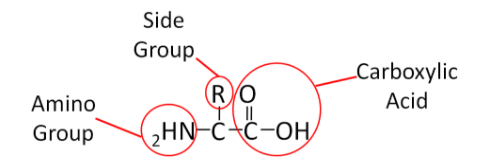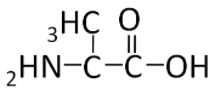# 2.2A: Amino Acids

•• Contributed by Brian Lindshield
• Associate Prof (Department of Food, Nutrition, Dietetics and Health) at Kansas State University

Similar to carbohydrates, proteins contain carbon (C), hydrogen (H), and oxygen (O). However, unlike carbohydrates (and lipids) proteins also contain nitrogen (N). Proteins are made up of smaller units called amino acids. This name, amino acid, signifies that each contains an amino (NH2) and carboxylic acid (COOH) groups. The only structural difference in the 20 amino acids is the side group represented by the R below.Fig $$\PageIndex{1}$$: Structure of an amino acid

To illustrate the differences in the side group we will consider glycine and alanine, the two simplest amino acids. For glycine the R group is hydrogen (H), while in alanine the R group is a methyl (CH3). The structures of these two amino acids are shown below.Figure $$\PageIndex{2}$$: Structure of glycineFigure $$\PageIndex{3}$$: Structure of alanine

Individual amino acids are joined together using a peptide bond (green) and is shown in the figure below.Figure $$\PageIndex{4}$$: Peptide bond formation1

Amino acids can also come together to form tripeptides (three amino acids), oligopeptides (3-10 amino acids), and polypeptides (10 or more amino acids). A polypeptide is a chain of amino acids as shown below.Figure $$\PageIndex{6}$$: A polypeptide chain2